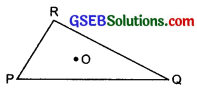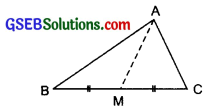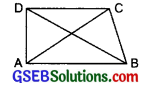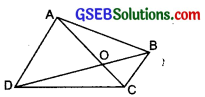# GSEB Solutions Class 7 Maths Chapter 6 The Triangles and Its Properties Ex 6.4

Gujarat Board GSEB Textbook Solutions Class 7 Maths Chapter 6 The Triangles and Its Properties Ex 6.4 Textbook Questions and Answers.

## Gujarat Board Textbook Solutions Class 7 Maths Chapter 6 The Triangles and Its Properties Ex 6.4Question 1.
Is it possible to have a triangle with the following sides?
(i) 2 cm, 3 cm, 5 cm
(ii) 3 cm, 6 cm, 7 cm
(iii) 6 cm, 3 cm, 2 cm
Solution:
(i) 2 cm, 3 cm, 5 cm
∵ 2 cm + 3 cm= 5 cm and the third side = 5 cm.
∴ Sum of the measures of lengths of two sides = length of third side which is not possible
i.e. A triangle cannot be possible with these sides.

(ii) 3 cm, 6 cm, 7 cm
∵ 3 cm + 6 cm = 9 cm and 9 cm > 7 cm
3 cm + 7 cm = 10 cm and 10 cm > 6 cm
6 cm + 7 cm = 13 cm and 13 cm > 3 cm
Thus, a triangle can be possible with these sides.

(iii) 6 cm, 3 cm and 2 cm
∵ 6 cm + 3 cm = 9 cm and 9 cm > 2 cm
3 cm + 2 cm = 5 cm and 5 cm > 6 cm
2 cm + 6 cm = 8 cm and 8 cm > 5 cm
∴ A triangle cannot be possible with these sides.Question 2.
Take any point O in the interior of a triangle PQR. Is
(i) OP + OQ> PQ?
(ii) OQ + OR > QR?
(iii) OR + OP > RP?Solution:
(i) Yes, OP + OQ > PQ
[ ∵ The sum of lengths of any two sides of a triangle is greater than the length of the third side.]
Similarly,
(ii) Yes, OQ + OR > QR
(iii) Yes, OR + OP > RP

Question 3.
AM is a median of a triangle ABC.
Is AB + BC + CA > 2AM? (Consider the sides of triangles ∆ABM and ∆AMC.)
Solution:
Since, the sum of the lengths of any two sides of a triangle is greater than the length of the third side.∴ In ∆ABM, we have
(AB + BM) >AM                       … (1)
Similarly, in ∆ACM,
(CA + CM) >AM                       … (2)
Adding (1) and (2), we have [(AB + BM) + (CA + CM)] > AM + AM
⇒ [AB + (BM + CM) + CA] > 2AM
⇒ [AB + (BC) + CA] > 2AM
Thus, (AB + BC + CA) > 2AM

Question 4.
Is AB + BC + CD + DA > AC + BD?Solution:
The sum of the lengths of any two sides of a triangle is greater than the length of the third side.
∵ In AABC, we have
(AB + BC) >AC                             … (1)
Similarly, in ∆ACD, we have
(CD + DA) >AC                            … (2)
Adding (1) and (2), we have [(AB + BC) + (CD + DA)] > 2AC … (3)
Again,
In ∆ABD, we have
AB + DA >BD … (4)
In ABCD, we have
BC + CD >BD … (5)
Adding (4) and (5), we have [(AB + DA) + (BC + CD)] > 2BD … (6)
Now, adding (3) and (6), we have
2[(AB + BC) + (CD + DA)] > 2(AC + BD) or (AB + BC + CD + DA) > (AC + BD)Question 5.
Is AB + BC + CD + DA < 2(AC + BD)? Solution: Since, the sum of the lengths of any two sides of a triangle is greater than the length of the third side.∴ In ∆OAB, we have (OA + OB) >AB … (1)
Similarly,
In ∆OBC, we have
(OB + OC) >BC … (2)
In AOCD, we have
(OC + OD) >CD … (3)
(OA + OD) >AD … (4)
Adding (1), (2), (3) and (4), we have 2[OA + OB + OC + OD] > (AB + BC + CD + DA)
⇒ (AB + BC + CD + DA) < 2[OA + OB + OC + OD]
⇒ (AB + BC + CD + DA) < 2[(OA + OC) + (OB + OD)]
⇒ (AB + BC + CD + DA) < 2[AC + BD]

Question 6.
The lengths of two sides of a triangle are 12 cm and 15 cm. Between what two measures should the length of the third side fall?
Solution:
Since, the sum of the lengths of any two sides of a triangle is greater than the length of the third side.
∴ Sum of 12 cm and 15 cm is greater than the length of the third side.
i.e. (12 cm + 15 cm) > (Third side)
i.e. 27 cm > Third side
or Third side < 27 cm
Also, the difference of the lengths of any two sides is less than the length of the third side.
∴ (15 cm – 12 cm) < Third side
or 3 cm < Third side.
Thus, we have 3 cm < Third side < 27 cm
∴ The third side should be any length between 3 cm and 27 cm.